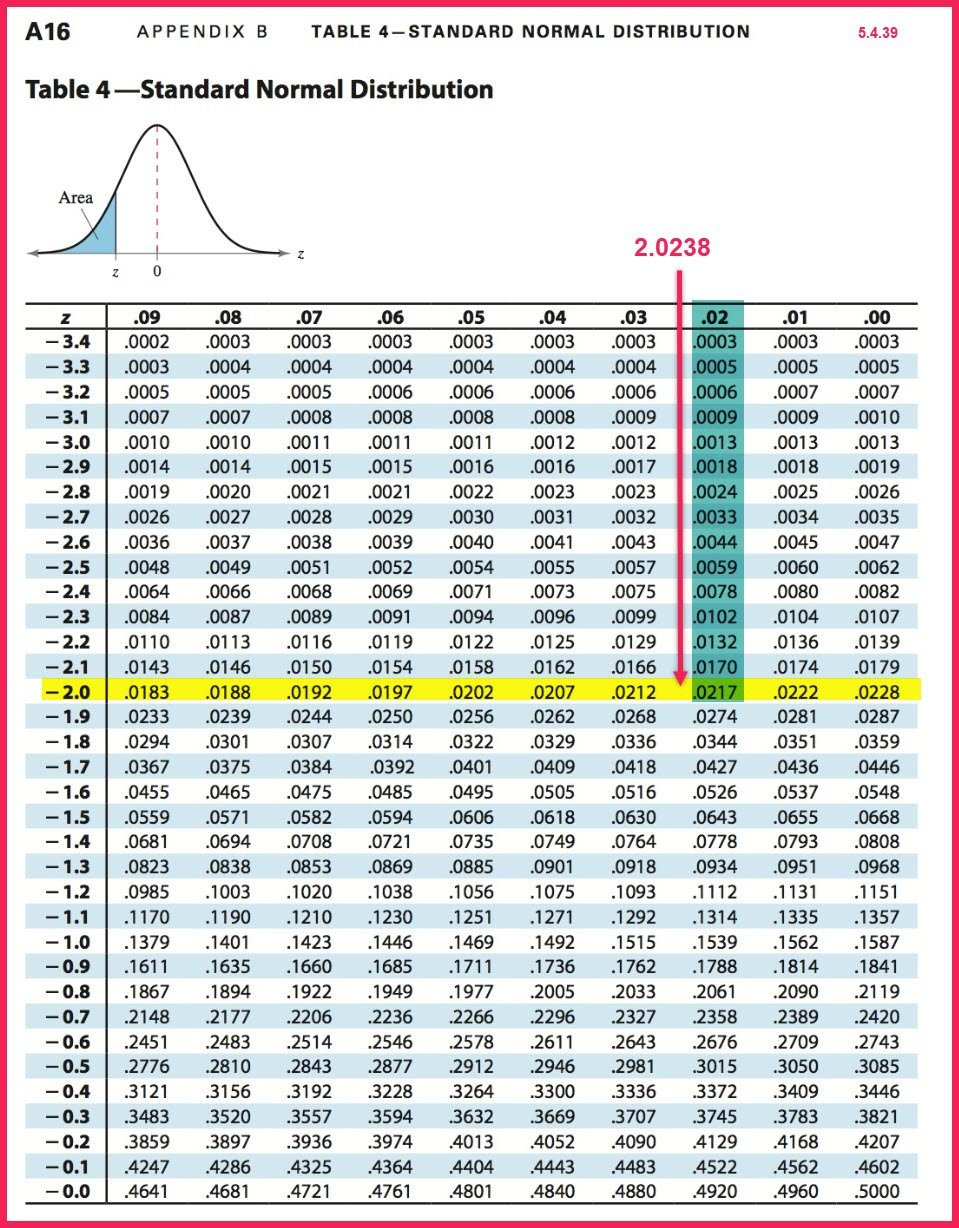# Technology vs Tables and Rounding Mistakes

Joe,

Two important points you bring up:

1. Do not round intermediate values in a string of calculations. Wait until the very last step/answer to round to the number of decimals required by MyStatLab. On this problem and many others in this course, rounding too early can lead to wrong answers.

In the instructor view, I can cycle through the variations of that problem different students might see. On about half, I could round the z value to two decimal places and still get the correct answer. But for the one shown, I get the wrong answer. On all of them, if I round the standard error, sigma x-bar, to three decimal places, I always got the wrong answer. Rounding early is tempting if you are using a calculator and writing down the intermediate values instead of storing them in the calculator’s memory, if that is possible for your calculator.1. All tables today are created using technology, not the reverse. So do not delude yourself into thinking the tables are more accurate. If you enter the normal table on this problem with the rounded z of -2.02, you will get the wrong answer unless you interpolate between the table values. Note: on some problems where MyStatLab does not tell you to use the tables, it may accept the nearest table value (intersection of highlights). But if the problem says, “Use technology,” the approximate table value will be counted wrong.(Larson & Farber, 2015, p. A16)

# References

Larson, R., & Farber, B. (2015). Elementary Statistics: Picturing the World, 6th. Boston: Pearson.

This site uses Akismet to reduce spam. Learn how your comment data is processed.